Courses

# Previous Year Questions: Fluid Mechanics

## 5 Questions MCQ Test Physics Class 11 | Previous Year Questions: Fluid Mechanics

Description
This mock test of Previous Year Questions: Fluid Mechanics for JEE helps you for every JEE entrance exam. This contains 5 Multiple Choice Questions for JEE Previous Year Questions: Fluid Mechanics (mcq) to study with solutions a complete question bank. The solved questions answers in this Previous Year Questions: Fluid Mechanics quiz give you a good mix of easy questions and tough questions. JEE students definitely take this Previous Year Questions: Fluid Mechanics exercise for a better result in the exam. You can find other Previous Year Questions: Fluid Mechanics extra questions, long questions & short questions for JEE on EduRev as well by searching above.
QUESTION: 1

### A cylinder of height 20 m is completely filled with water. The velocity of efflux of water (in ms-1) through a small hole on the side wall of the cylinder near its bottom, is [AIEEE 2002]

Solution: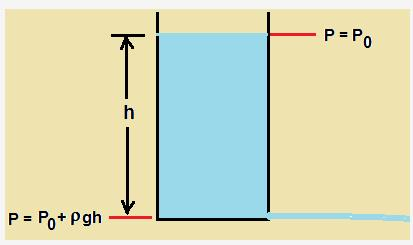As per bernauli's equation  p+(1/2)ρu2 is constant.

If we apply the bernauli's equaition at the top surface and at the bottom hole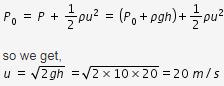QUESTION: 2

### A jar is filled with two non-mixing liquids 1 and 2 having densities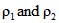respectively. A solid ball, made of a material of density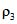, is dropped in the jar. It comes to equillibrium in the position shown in the figure. Which of the following is true for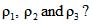[AIEEE 2008]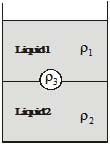Solution:

The density of ball should be greater than liquid 1 because it sinks in it.
Also density of ball should be less than density of liquid 2 as it floats on it.

QUESTION: 3

### A ball is made of a material of densityr where,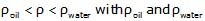representing the densities of oil and water, respectively. The oil and water are immiscible. If the above ball is in equilibrium in a mixture of this oil and water, which of the following pictures represents its equilibrium positions? [AIEEE 2010]

Solution:

As density of ball is more than that of oil, so, it remain sunk in the oil. Therefore option A and option D are wrong as ball is floating on the oil. Now for option B, as density of water is more than that of oil, so, it remains under the oil which is in case of option B.

QUESTION: 4

Water is flowing continuously from a tap having an internal diameter 8 × 10-3m. The water velocity as it leaves the tap is 0.4 ms-1. The diameter of the water stream at a distance 2 × 10-1 m below the tap is close to

[AIEEE 2011]

Solution: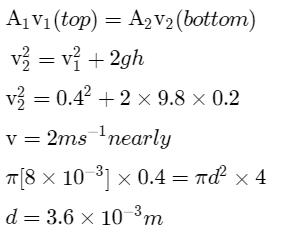QUESTION: 5

A uniform cylinder of length L and mass M having cross - sectional area A is suspended, with its length vertical, from a fixed point by a massless spring, such that it is half submerged in a liquid of density s at equilibrium position. The extension x0 of the spring when it is in equilibrium is :

[JEE Main 2013]

Solution: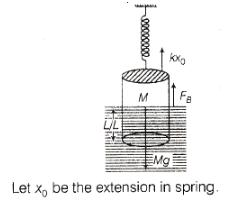where, k is spring constant, FB is force due to buoyancy which is equal to weight of liquid displaced.
FB= σ.L.A.g /2
kx0 + σ.L.A.g /2=Mg
x0=Mg-σ.L.A.g /2/k=Mg/k(1- σ.L.A/2M)